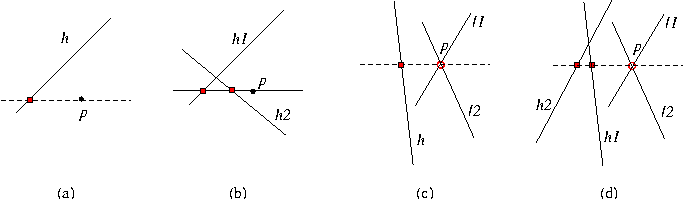CGAL::compare_x_at_y

Comparison_result compare_x_at_y ( Point_2<Kernel> p, Line_2<Kernel> h)
compares the x-coordinates of p and the horizontal projection of p on h; see (a) in the figure below.
 Precondition: h is not horizontal.

Comparison_result compare_x_at_y ( Point_2<Kernel> p, Line_2<Kernel> h1, Line_2<Kernel> h2)
This function compares the x-coordinates of the horizontal projection of p on h1 and on h2; see (b) in the figure below.
 Precondition: h1 and h2 are not horizontal.

Comparison_result compare_x_at_y ( Line_2<Kernel> l1, Line_2<Kernel> l2, Line_2<Kernel> h)
Let p be the intersection of lines l1 and l2. This function compares the x-coordinates of p and the horizontal projection of p on h; see (c) in the figure below.
 Precondition: l1 and l2 intersect and are not horizontal; h is not horizontal.

Comparison_result compare_x_at_y ( Line_2<Kernel> l1, Line_2<Kernel> l2, Line_2<Kernel> h1, Line_2<Kernel> h2)
Let p be the intersection of lines l1 and l2. This function compares the x-coordinates of the horizontal projection of p on h1 and on h2; see (d) in the figure below.
 Precondition: l1 and l2 intersect and are not horizontal; h1 and h2 are not horizontal.